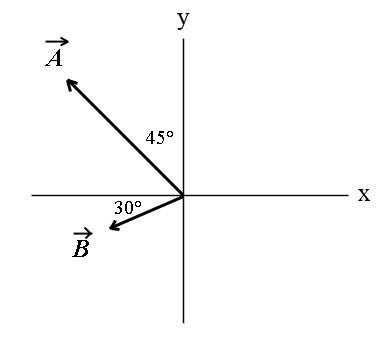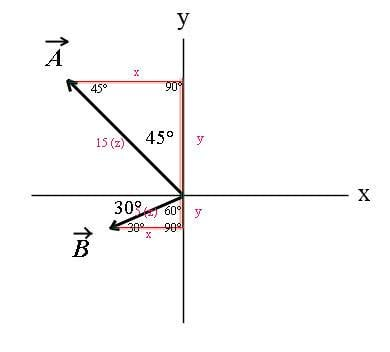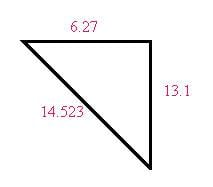# Find the vector and find the magnitude of the vector

## Homework Statement

Vectors A and B are shown in the figure. Vector C is given by C=B-A. The magnitude of vector A is 15.0 units, and the magnitude of Vector B is 5.00 units.Part a) Find the vector C?

Part b) What is the magnitude of vector C?

SOHCAHTOA

## The Attempt at a Solution

I drew these lines on the diagram and then solve for the sides using the angles with the SOHCAHTOA Method. Then after I found the sides I use the Pythagorean theorem to solve for side C. I am assuming vector C is the line connected with vector A and B.Part b) the magnitude of vector C is 14.523.

Q: How to do part a? I don't understand what it means by finding vector C. Also is the above correct?

Simon Bridge
Homework Helper
finding vector C is what you did when you worked out the components. You cannot do part (b) without it. You can answer the question by drawing the vector on the diagram and/or by writing out the components with the i and j unit vectors.

Did you see that the two vectors use special triangles so you don't need a calculator?

finding vector C is what you did when you worked out the components. You cannot do part (b) without it. You can answer the question by drawing the vector on the diagram and/or by writing out the components with the i and j unit vectors.

Did you see that the two vectors use special triangles so you don't need a calculator?

I'm a bit confused.

The value I got, 14.523 units, is that the answer for part b, which is to find the magnitude?

So if I understand what you're saying, part a is to either draw the vector on the diagram or write out the components. Example answer is Vector C = 6.27i + 13.1j units.

Simon Bridge
Homework Helper
Example answer is Vector C = 6.27i + 13.1j units.
That's a vector all right - well done! The magnitude of that vector would be 14.523 units... the answer to (b).

Hey quick question, how do I solve this using the physics method and not the pre-calculus method like I did above using SOHCAHTOA?

Simon Bridge
Homework Helper
sohcahtoa is just a mnemonic to remember the basic trigonometric relations.

trigonometry (the art of measuring triangles) is a basic tool of physics ... there is more to it than sohcahtoa.

In this case I think I suggested the special triangles.
The two in the diagram were 45-45-90, and 30-60-90 ...
The first one is the triangle you get when O=A, and the second is when H=2O
The lengths of the sides have ratios O:A= 1:1:√2 and 1:√3:2

i.e. when O=A then H=O√2

So
- for vector A: Ay=-Ax=|A|/√2
- for vector B: By=-|B|/2, Bx=-|B|(√3/2)

One advantage of this approach is that you can write:
|A|/√2 = 15/√2

... and stop there - it's exact: no need to go into decimals.
Leave the heavy calculator work to the end avoids accumulating rounding-off errors.
It also makes your working easier to troubleshoot if you make a mistake.

Another approach is to draw out the vectors head-to-tail so C=B-A = B+(-A)
the magnitude of C is the length of the third side of the resulting triangle - which you can get from the cosine rule. The sine rule gives you the other angles - which gives you the direction of C.
However - it is easiest to do this one in terms of components.